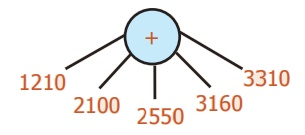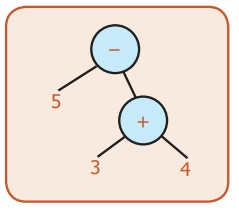Home | | Maths 6th Std | Exercise 5.2

# Exercise 5.2

6th Maths : Term 2 Unit 5 : Information Processing : Exercise 5.2 : Miscellaneous practice problems, Challenge problems, Text Book Back Exercises Questions with Answers, Solution

Exercise 5.2

Miscellaneous practice problems

1. Write the missing numbers in the trees.2. Write the missing operations in the trees.3. Check whether the Tree diagrams are equal or not.(a÷b)÷c    not equal to   a÷(b÷c)

Challenge problems

4. Convert the following questions into tree diagrams:

(i) The number of people who visited a library in the last 5 months were 1210, 2100, 2550, 3160 and 3310. Draw the tree diagram of the total number of people who had used the library for the 5 months.1210 + 2100 + 2550 + 3160 + 3310 = 12330

(ii) Ram had a bank deposit of Rs. 7,55,250 and he had withdrawn Rs.5,34,500 for educational purpose. Find the amount left in his account. Draw a tree diagram for this.7,55,250 − 5,34,500 = 2,20,750

(iii) In a cycle factory, 1,600 bicycles were manufactured on a day. Draw tree diagram to find the number of bicycles produced in 20 days.1600 × 20 = 32,000

(iv) A company with 30 employees decided to distribute Rs.90,000 as a special bonus equally among its employees. Draw tree diagram to show how much will each receive?90000 ÷ 30 = 3000

5. Write the numerical expression which gives the answer 10 and also convert into tree diagram.5 × 2 = 10

6. Use brackets in appropriate place to the expression 3 × 8 − 5 which gives 19 and convert it into tree diagram for it.(3 × 8) – 5 = 19

7. A football team gains 3 and 4 points for successive 2 days and loses 5 points on the third day. Find the total points scored by the team and also represent this in tree diagram.(3 + 4) – 5 = 2

Exercise 5.2

1)2)3) Not equal

4)7)Tags : Questions with Answers, Solution | Information Processing | Term 2 Chapter 5 | 6th Maths , 6th Maths : Term 2 Unit 5 : Information Processing
Study Material, Lecturing Notes, Assignment, Reference, Wiki description explanation, brief detail
6th Maths : Term 2 Unit 5 : Information Processing : Exercise 5.2 | Questions with Answers, Solution | Information Processing | Term 2 Chapter 5 | 6th Maths